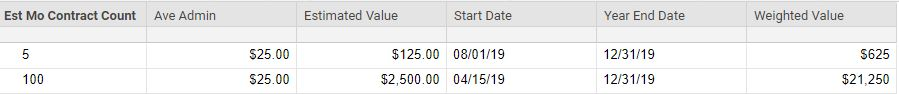# Formula needed that will generate quantity in one column against date in another column = how much a

I have the following columns and need to return the value of something from a date through the end of the year.

1. Est Mo Contract Count - A number

2. Ave admin - Dollar Amount

3. Estimated Value - 1 x 2

4. Start Date - Start Date that 1 actually goes into effect

5. Weighted Value - Sum of 4 through end of the current year (12/31/2019).

Example 1

1 = 5 (Est Mo Contract Count)

3 = \$125 (5 x \$5) (Estimated Value)

4 = 8/1/2019 (Start Date that #1 actually goes into effect & 4 months remaining in year)

5 = \$625 (\$125 x 4 months) (Weighted Value)

Example 2

1 = 100 (Est Mo Contract Count)

3 = \$2500 (100 x \$25) (Estimated Value)

4 = 4/15/2019 (Start Date that #1 actually goes into effect & 8.5 months remaining in year)

5 = \$21,250 (\$2500 x 8.5 months) (Weighted Value)

I'm sure this is more simple than what I can think up.

Thank you very much for reviewing, I appreciate it very much.

Tags:

• Someone will probably come along with an embarassingly easier way to do it, but here's what I came up.  If the start date is the 15th or later, it counts as 1/2 of a month.  earlier than the 15th, a full month.

=[Estimated Value]2 * ((MONTH([Year End Date]2) - MONTH([Start Date]2)) + IF(DAY([Start Date]2) > 14, 0.5, 1))• David Hersher,

Thank you so very much for your help with this formula. It works perfectly.

Have a great day!

Angie Hatfield

• Depending on how accurate you are wanting to make the weight, you could also use this to weight it down to the day...

=[Estimated Value]@row * (12 - ((YEARDAY(DATE(YEAR([Start Date]@row), 12, 31)) - YEARDAY([Start Date]@row)) / YEARDAY(DATE(YEAR([Start Date]@row), 12, 31))))

The weight for today (24 Jan. 2019) would be 11.06575

Totally unnecessary if you are fine with going by the half month. It just piqued my curiosity as to how specific I could get it. Haha

• Paul Newcome,

Although my VP of Sales was very happy with half month calc, she will like this even better.

It works great. Thank you very much for being inquisitive!

Angie Hatfield

## Help Article Resources

Want to practice working with formulas directly in Smartsheet?

Check out the Formula Handbook template!